# Dice Reasoning Practice Questions: Level 02

Q.1. A dice with six faces is marked with six numbers 1, 2, 3, 4, 5  and 6 respectively. This dice is rolled three times and three positions are shown as: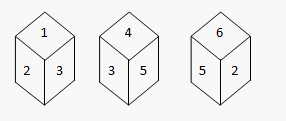Find the number opposite to 1.
A. 2
B. 6
C. 5
D. 4
Q.2. You are given three positions of dice then which face is opposite to the face with alphabet B?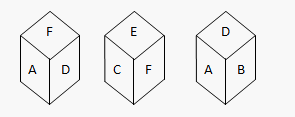A. E
B. F
C. D
D. A
Q.3. Which face is opposite to face with alphabet B, if four positions are given as below as?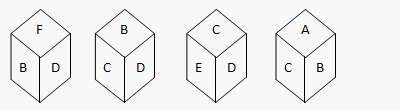A. B
B. A
C. F
D. E
Q.4. Which of the following patterns can be formed from the piece of cardboard (X) as shown below?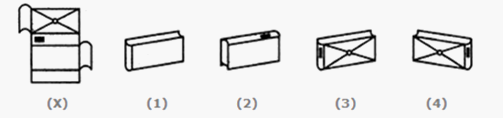A. 4
B. 2
C. 1
D. 3
Directions for questions 5 to 9: Six different positions of dice are given below: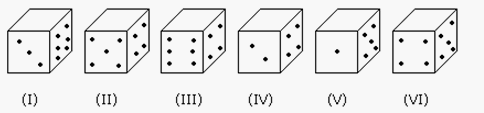The sum of the numbers of dots on the opposite face is 7.
Q.5. If an even numbered dice have an odd number of dots on their top faces, then find the total number of dots on the top faces of their dice?
A. 22
B. 3
C. 10
D. 24
Q.6. If an odd numbered dice have an odd number of dots on their top faces, then find the total number of dots on top faces of their dice is?
A. 11
B. 12
C. 13
D. 14
Q.7. . If dice (I), (II) and (III) have an odd number of dots on their top faces, and the dice (IV), (V) and (VI) have an even number of dots on their top faces, then what is the difference in the total number of top faces between the two sets?
A. 0
B. 3
C. 1
D. -5
Q8. If the odd numbers of dice have an even number of dots on their top faces and an even numbered dice have an odd of dots on their bottom faces, then what is the total number of dots on their top faces?
A. 26
B. 12
C. 16
D. 18
Q9. If the dice (I), (II) and (III) have an odd number of dots on their top faces, then what is the total number of dots on their top faces?
A. 7
B. 14
C. 12
D. 11
Q10.Refer to the following four positions of the dice and find out the color which is opposite the face grey?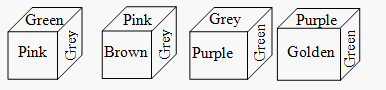A. Golden
B. Purple
C. Brown
D. Green